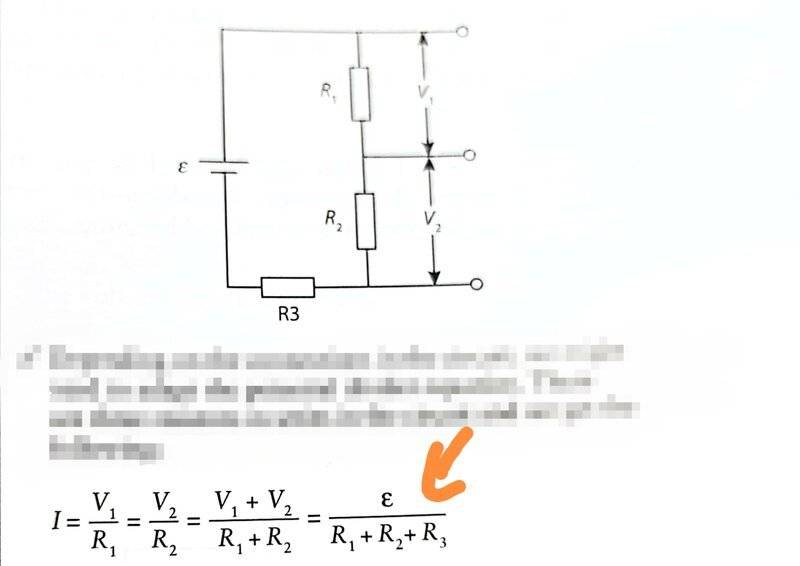• ellieee
ellieee
Homework Statement
I'm not sure what this symbol means
Relevant Equations
V1 = R1 / R1+R2+R3 x "inverted 3"Last edited by a moderator:

phinfinity
The epsilon symbol (##\epsilon##) stands for "emf" or ElectroMotive Force. I think it's a bit of a technicality to name voltage sources like batteries with ##\epsilon## while potential difference generated across other components are labeled as Voltage (V).

•Steve4Physics, ellieee and Delta2
Homework Helper
Gold Member
2022 Award
The epsilon symbol (##\epsilon##) stands for "emf" or ElectroMotive Force. I think it's a bit of a technicality to name voltage sources like batteries with ##\epsilon## while potential difference generated across other components are labeled as Voltage (V).
I know I'm being pedantic but can I add:

1) It is ##\mathscr E## not an epsilon (##\epsilon##). The symbol is a 'script E'. My school physics teacher used to call it a 'curly E'. (To get the symbol with Latex, use \mathscr E.)

2) There is an important difference between the voltage (more correctly terminal pd), V, of a voltage-source and the source's emf ##\mathscr E##.

If the voltage-source has some internal resistance, then when a current flows V is smaller than ##\mathscr E##. But for an ideal voltage-source (zero internal resistance) V and ##\mathscr E## are the same value. Most simple circuit problems assume an ideal voltage-source; in that case you don't need to worry about the difference.

•Delta2 and ellieee